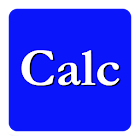Calculator

All Android applications categories

All Android games categories# Calculator

4 9.6

9.6 Users
rating

## Screenshots

Description

Calculator is the complete RPN calculator for Android.

It includes

* reverse polish notation calculator with about 100 functions (standard, trigonometric, logarithmic, hyperbolic, matrix, logic, number theory, ...)
* unit-conversion and calculation (us, imperial and international unit system)
for example: enter 5 foot, enter 4 inches, multiply and you will get 1.66 sq.ft
supports SI-prefixes: Enter 5 volt, enter 200 micro ampere and divide them! (Hint: Its's 25 kΩ)
define your own units and use them just like system units. Ideal for engineering!
* complex numbers in algebraic or exponential display mode (argument in radian or degree)
Mix them with real numbers in two argument functions (add, div, ...)
See and enter the argument in radian or degrees, depending on the trigonometric mode
Please be aware of the complex mode when entering data; don't assume it is always a+bi !
* (large) integer numbers with
logic (and, or, not, xor, shift, rotate, ...) and
number theory functions (modulo, gcd, n!, gamma function, binomial coefficients, factorizing numbers, ...)
use them in (most of) the existing functions.
logic and some arithmetic functions unbounded or with a fixed word length
* full support of matrix (and vector) operations plus
determinant, trace, transposition, inverse, power
matrix norms: column sum norm, row sum norm, spectral norm, frobenius norm, maximum norm
LU- and QR-decomposition (inspect L,U and Q,R if you want) for solving linear equations
Eigen values and eigen vectors of a matrix
Matrix functions: Matrix exponential (pade approximation)
* data transfer to other devices
easily transfer all your data to other devices. No need to re-enter everything when changing devices.

It is easy to use

* assign the buttons the functions you want with a simple swipe of your finger!
* record macros to simplify often used routines
* extensive built-in help
* permanent register storage for your constants etc.
* transfer your data to another device
* full undo functionality
* no limits on stack or register storage
* full support of degree-minutes-seconds formats (including calculation!)
* number systems: floating point or integer
* number formats: four floating point formats as well as decimal, binary, octal and hexadecimal for integers.

Have a look at the definition of a function or conversion by clicking the entry in help->list of functions/conversions.

from 4 reviews

"Awesome"

9.6